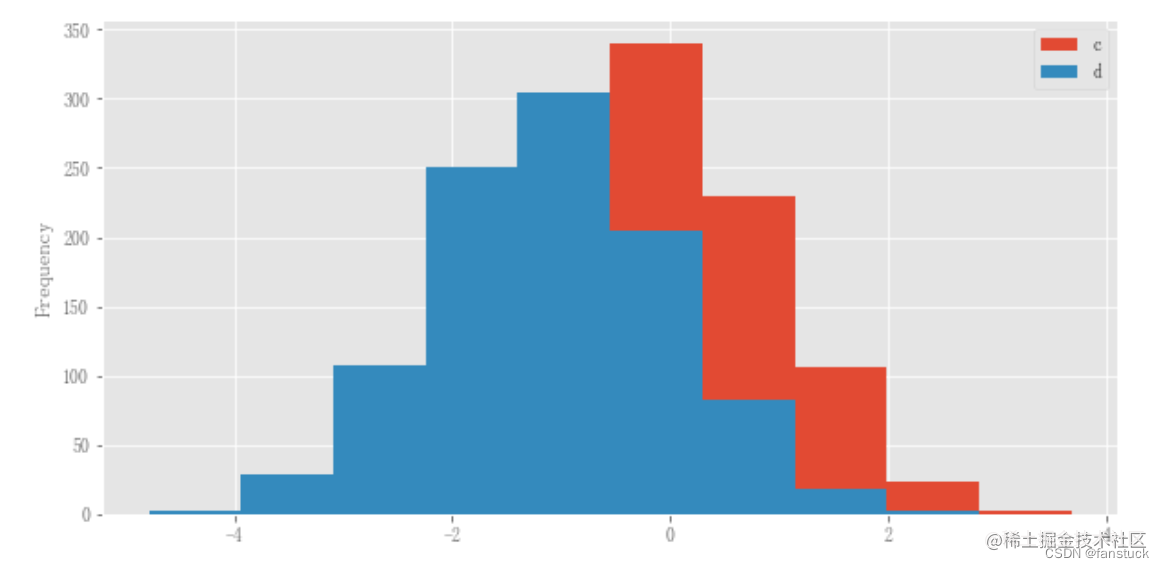# 前言

Pandas數據分析系列專欄已經更新了很久了，基本覆蓋到使用pandas處理日常業務以及常規的數據分析方方面面的問題。從基礎的數據結構逐步入門到處理各類數據以及專業的pandas常用函數講解都花費了大量時間和心思創作，如果大家有需要從事數據分析或者大數據開發的朋友推薦訂閲專欄，將在第一時間學習到Pandas數據分析最實用常用的知識。此篇博客篇幅較長，涉及到處理文本數據(str/object)等各類操作，值得細讀實踐一番，我會將Pandas的精華部分挑出細講實踐。博主會長期維護博文，有錯誤或者疑惑可以在評論區指出，感謝大家的支持。

# 一、基礎繪圖：plot

Series和DataFrame上的plot方法只是plt.plot（）的簡單包裝,這裏我們用一段實際數據來進行可視化展示：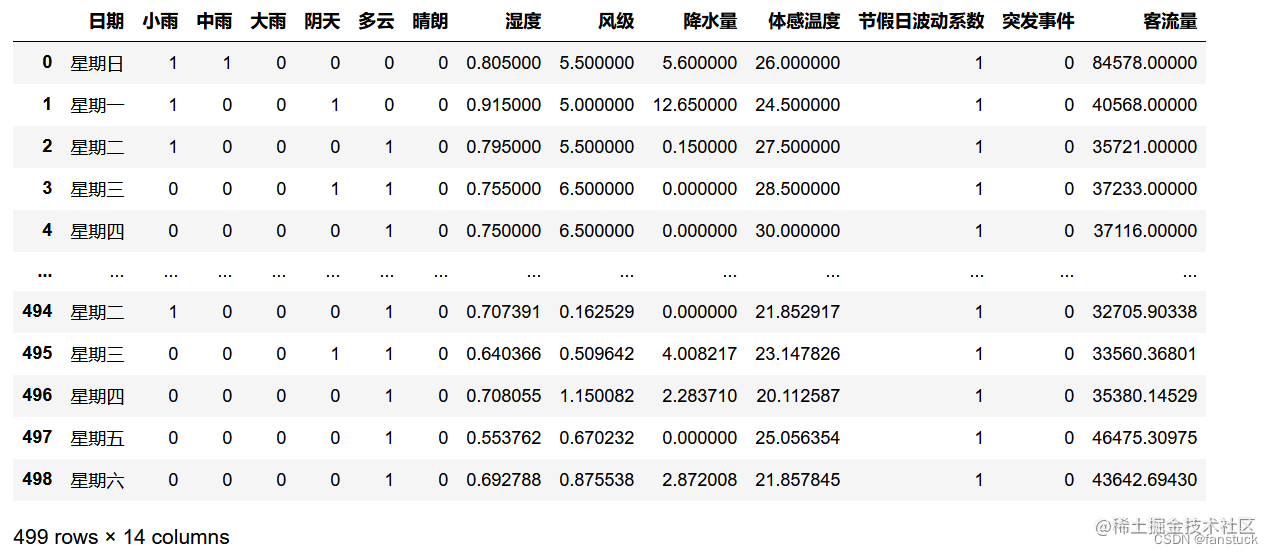`df_flow['客流量'].plot()`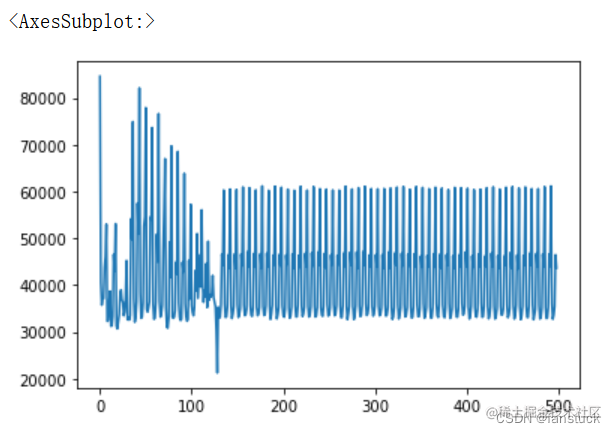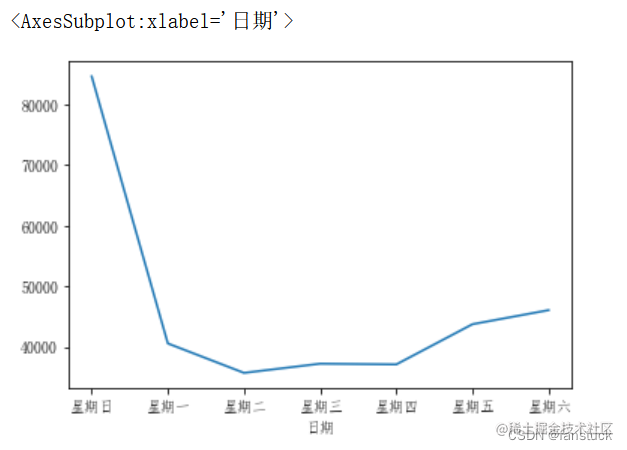`df_flow_mark[['濕度','風級','降水量']].plot()`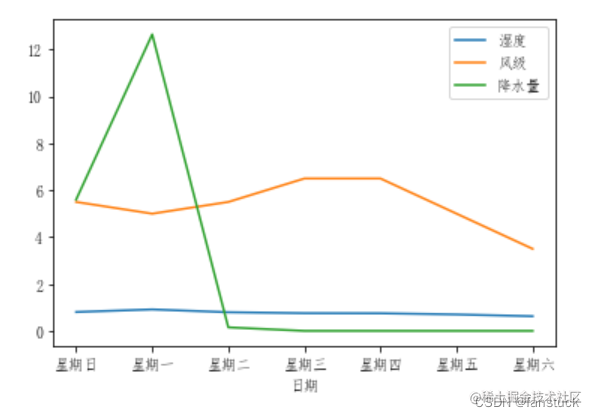```df_flow_7=df_flow[df_flow['日期']=='星期日'].iloc[:7,:] df_flow_7.rename(columns={'客流量':'星期日客流量'},inplace=True) df_flow_6=df_flow[df_flow['日期']=='星期六'].iloc[:7,:] df_flow_6.rename(columns={'客流量':'星期六客流量'},inplace=True) df_compare=pd.concat([columns_convert_df(df_flow_7['星期日客流量']),columns_convert_df(df_flow_6['星期六客流量'])],axis=1) df_compare.plot(x='星期日客流量',y='星期六客流量')```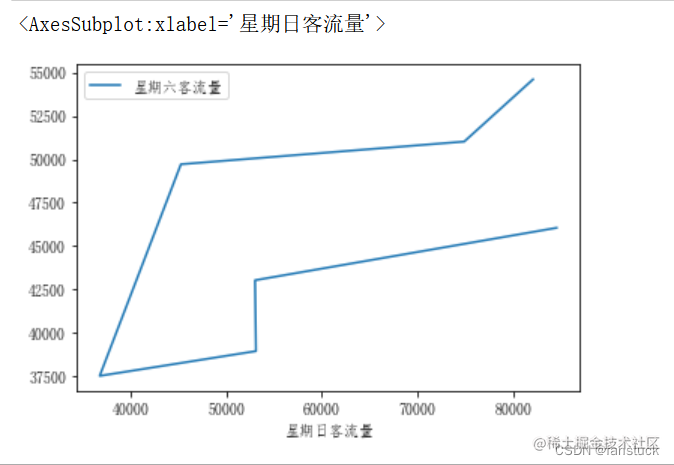# 二、底圖板塊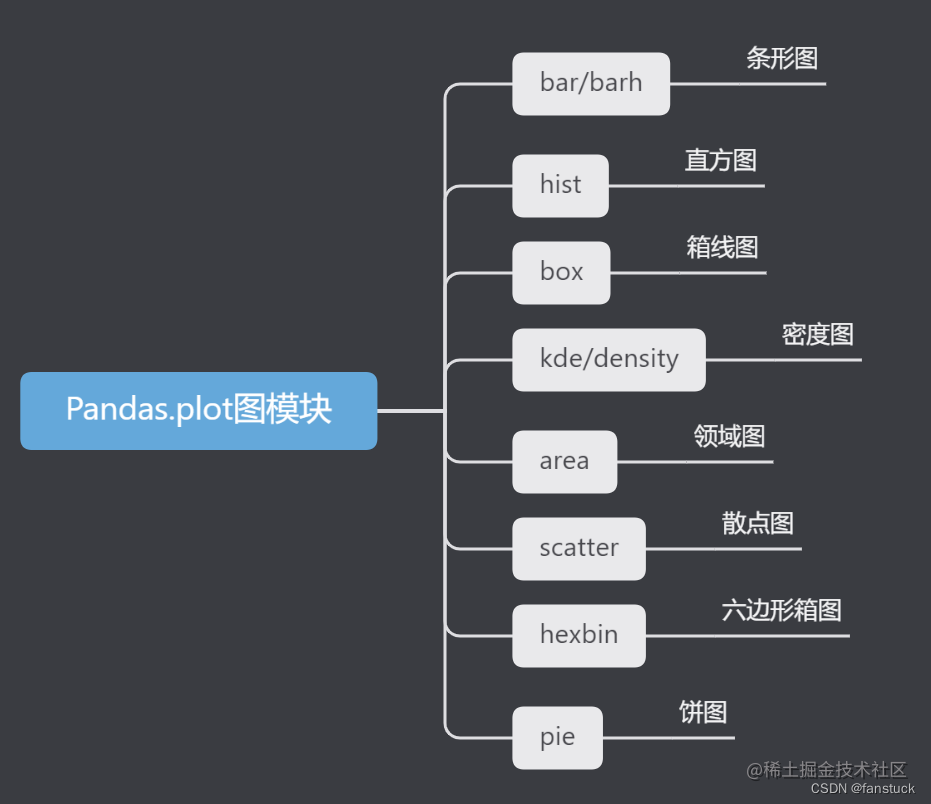``` df.plot.area df.plot.barh df.plot.density df.plot.hist df.plot.line df.plot.scatter df.plot.bar df.plot.box df.plot.hexbin df.plot.kde df.plot.pie

``` 除了這些類型，還有DataFrame.hist（）和DataFrame.boxplot（）方法，它們使用單獨的接口。

## 1.條形圖

`df_flow_mark['客流量'].plot(kind='bar')`

`df_flow_mark['客流量'].plot.bar()`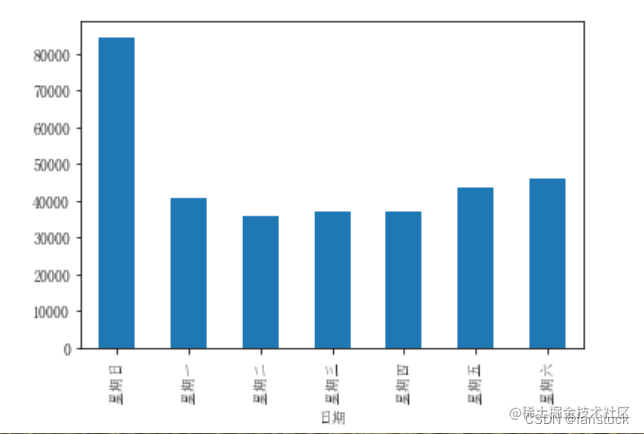`df_flow_mark[['風級','降水量']].plot.bar()`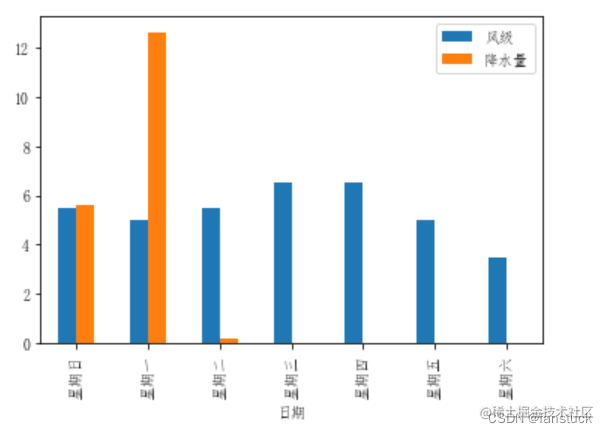`df_flow_mark[['風級','降水量']].plot.bar(stacked=True)`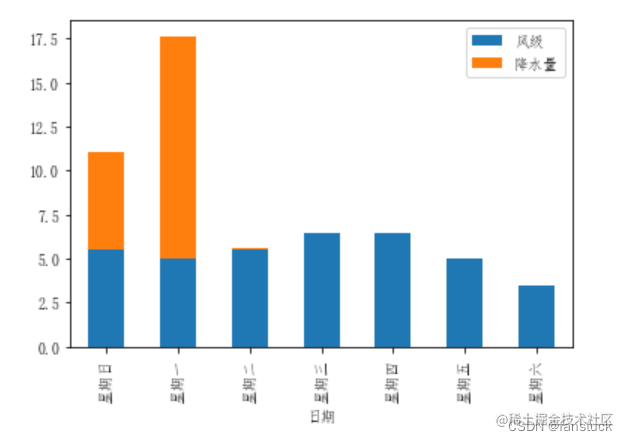`df_flow_mark[['風級','降水量']].plot.barh(stacked=True)`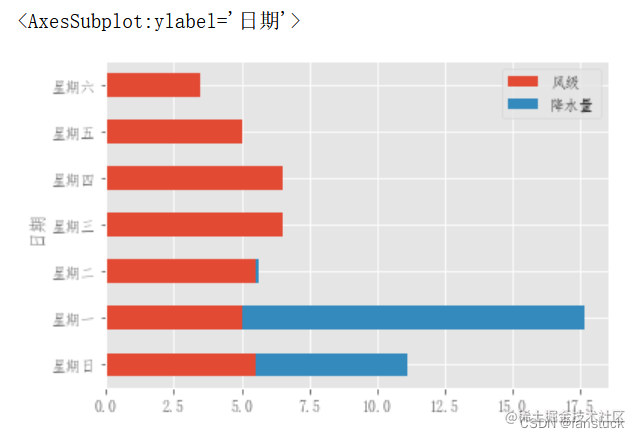## 2.直方圖

```df4 = pd.DataFrame( { "a": np.random.randn(1000) + 1, "b": np.random.randn(1000), "c": np.random.randn(1000) - 1, }, columns=["a", "b", "c"], ) plt.figure(); df4.plot.hist(alpha=0.5)```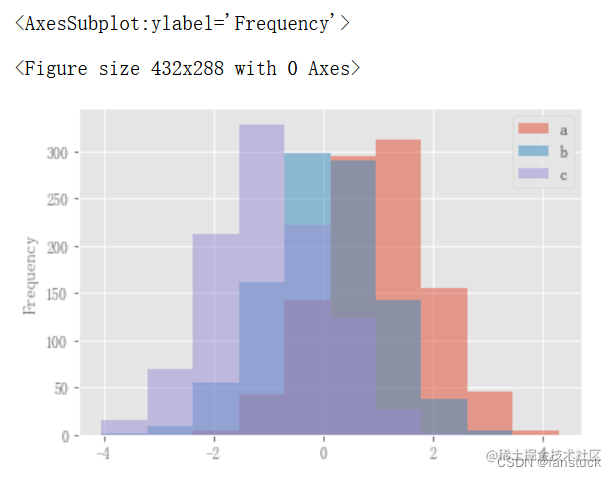直方圖可以使用stacked=True進行疊加。可以使用bins關鍵字更改bin大小。

`df4.plot.hist(stacked=True, bins=20);` 可以傳遞matplotlib hist支持的其他關鍵字。例如，水平和累積直方圖可以通過`orientation='horizontal'``cumulative=True`繪製。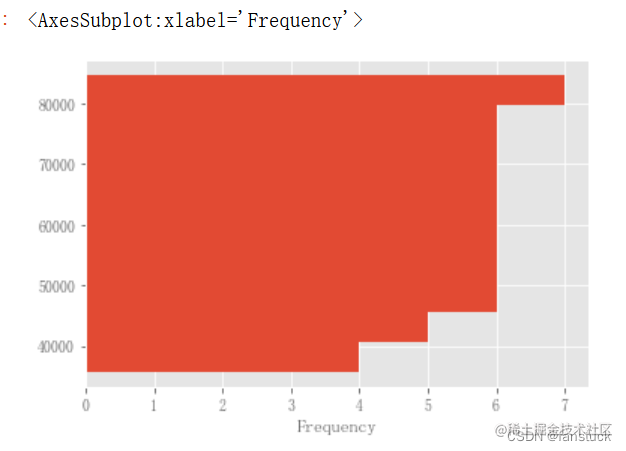```plt.figure(); df_flow_mark['風級'].hist();```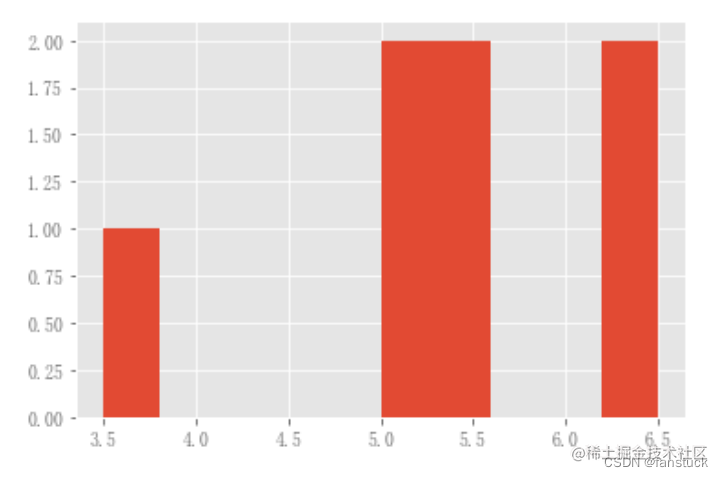DataFrame.hist()可以在多個子地塊上繪製列的直方圖：

```plt.figure(); df_flow_mark[['風級','降水量']].diff().hist(color="k", alpha=0.5, bins=50);```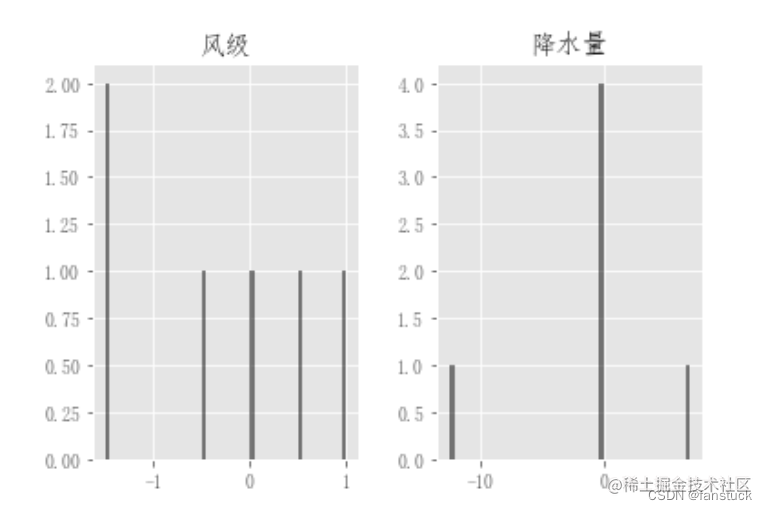``` data = pd.Series(np.random.randn(1000))

data.hist(by=np.random.randint(0, 4, 1000), figsize=(6, 4)); ```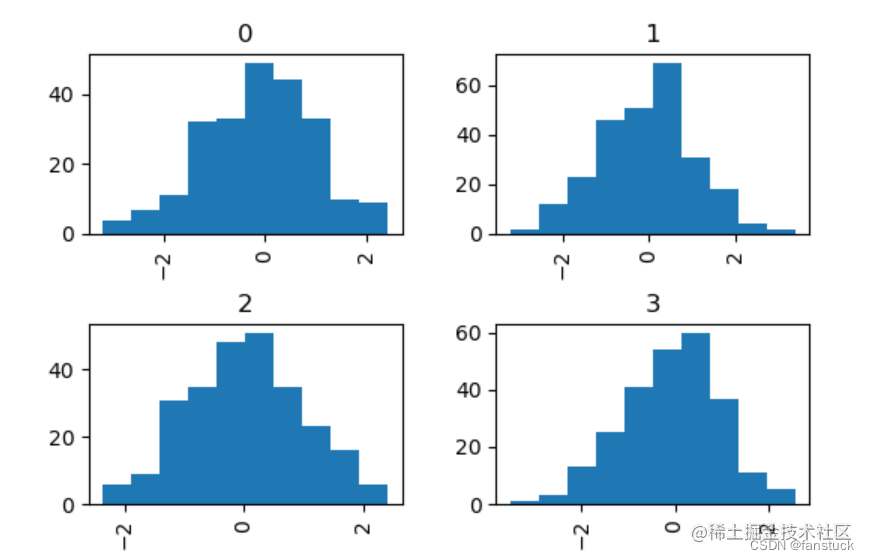```data = pd.DataFrame( { "a": np.random.choice(["x", "y", "z"], 1000), "b": np.random.choice(["e", "f", "g"], 1000), "c": np.random.randn(1000), "d": np.random.randn(1000) - 1 } ) data.plot.hist(by=["a", "b"], figsize=(10, 5));```